## Completion Of Incomplete Pattern

#### Completion Of Incomplete Pattern

Direction: In each of the following questions which one of the answer figures shall complete the given question figure.

1. NA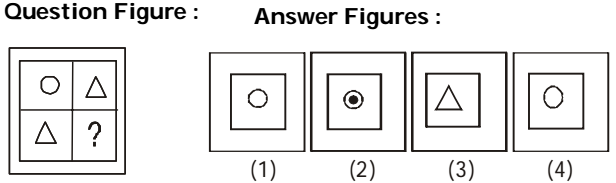1. In the given figure two triangles are arranged diagonally and hence one circle would complete the diagram.

##### Correct Option: A

In the given figure two triangles are arranged diagonally and hence one circle would complete the diagram.

1. NA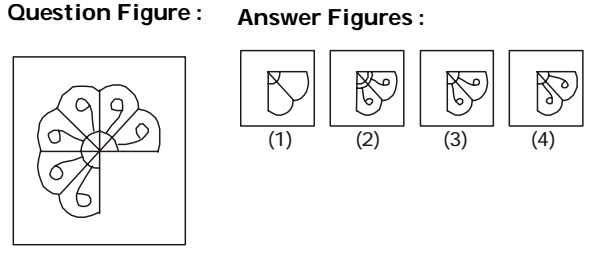1. NA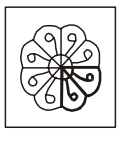##### Correct Option: C

NA1. NA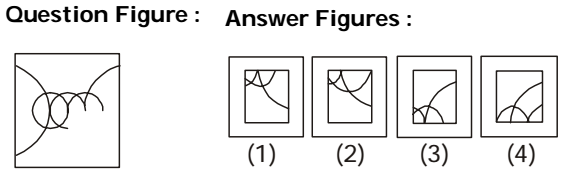1. NA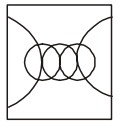##### Correct Option: A

NA1. NA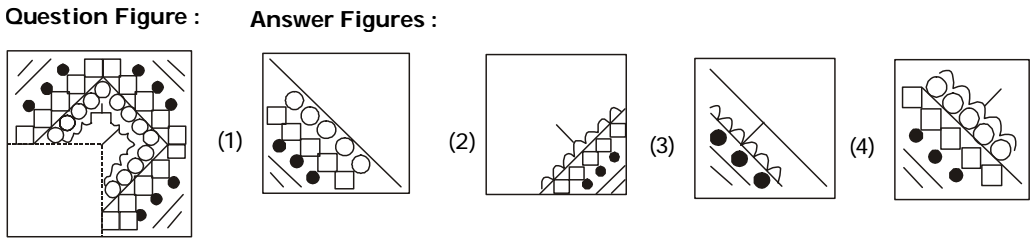1. NA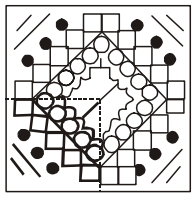##### Correct Option: D

NA1. NA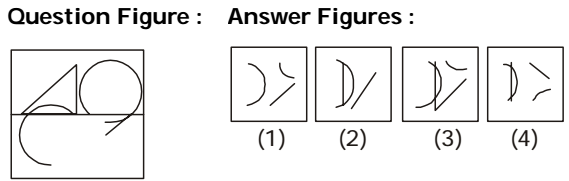1. NA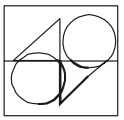##### Correct Option: C

NA